• NSGA2 约束条件处理的方法，包括非线性约束。NSGA2
• ## 遗传算法4约束条件

千次阅读 2020-05-04 17:46:11
matlab调用遗传算法 解释：fitnessfcn为函数名，nvars为自变量个数。A，b为AX<=b中的矩阵、向量。Aeq为等式约束矩阵，beq为等式约束矩阵对应的值向量，AeqX=beq，LB为变量下界,UB为变量上界。nonlcon为非线性约束...
matlab调用遗传算法
解释：fitnessfcn为函数名，nvars为自变量个数。A，b为AX<=b中的矩阵、向量。Aeq为等式约束矩阵，beq为等式约束矩阵对应的值向量，AeqX=beq，LB为变量下界,UB为变量上界。nonlcon为非线性约束，
exitflag表示过程收敛，粗略说来，-1和-2两种情况属于有异常的结束，即没找到最优解。1-5的情况都是在某种准则下收敛了，可以认为找到了（局部）最优解。0的情况是迭代次数很多但仍不收敛，这时可能离最优解还有一定距离。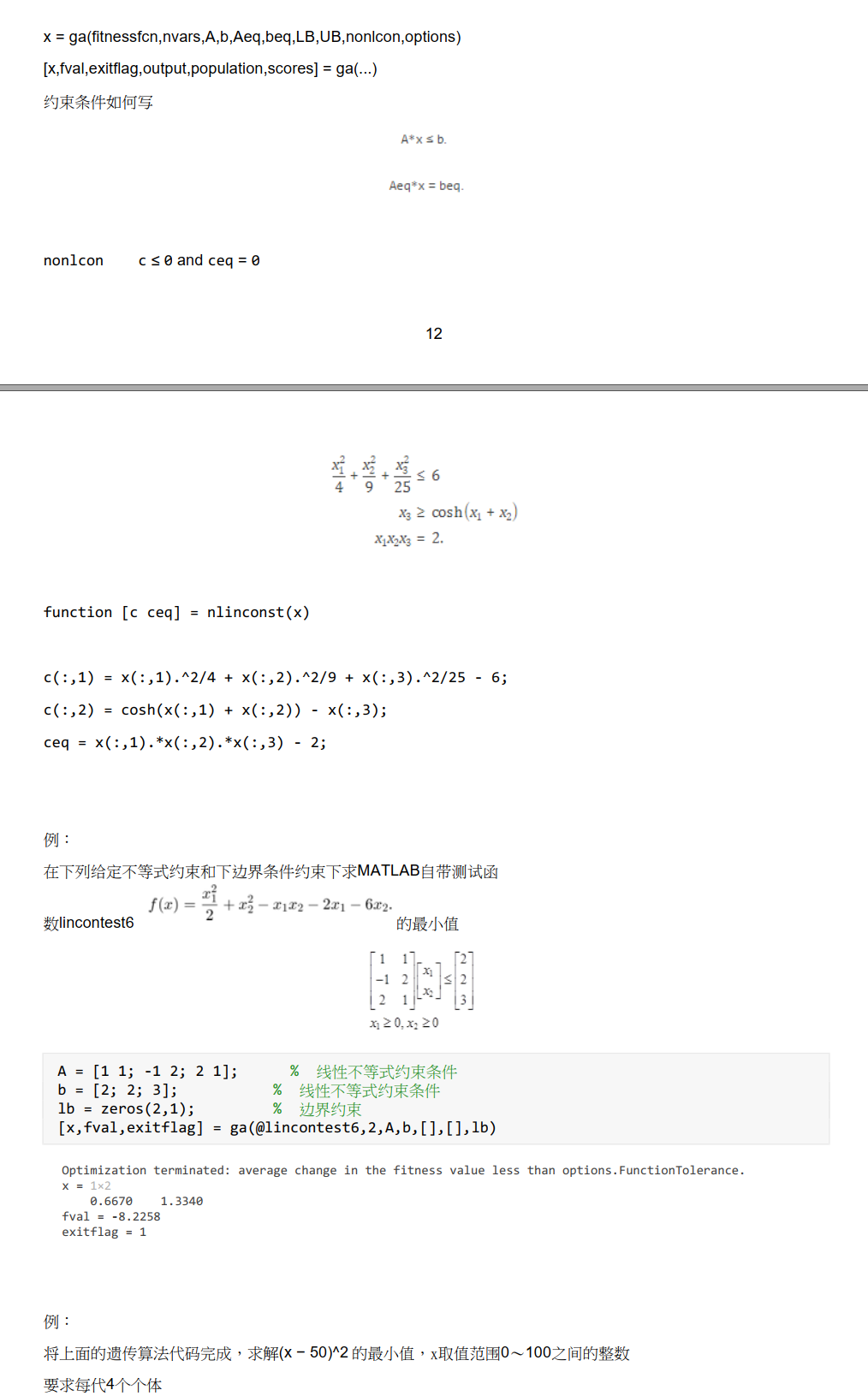展开全文matlab
• 带有约束条件遗传算法MATLAB源程序 带有约束条件遗传算法MATLAB源程序 带有约束条件遗传算法MATLAB源程序 带有约束条件遗传算法MATLAB源程序
• 遗传算法是计算数学中用于解决最佳化的搜索算法，是进化算法的一种，本文档以无约束条件的案例形式，讲解遗传算法
• 刚刚学习遗传算法 解决简单的一个非线性规划的问题(我把我的问题简化了） max z=x(1).^2+x(2).^2; 约束条件是x（1）+x(2)；...请问这两个约束条件如何写入我的遗传算法的代码中呢？应该写入遗传算法过程的哪个部分？人工智能 机器学习 深度学习 神经网络
• 研究了不同约束条件下，车辆...针对车辆调度模型设计了自然数编码的遗传算法，并改进了遗传算法中的交叉算法，尽可能地保留了最优基因的遗传性，加速了算法的收敛性。最后进行算例分析，结果表明了模型和算法的有效性。车辆调度问题
• 基于多约束条件遗传算法的雷达网优化部署.pdf 基于多约束条件遗传算法的雷达网优化部署.pdf
• %距离矩阵，满足三角关系，暂用距离表示花费c[i][j]=dist[i][j] %% 遗传算法参数设置 alpha=100000; %违反的容量约束的惩罚函数系数 belta=90;%违反时间窗约束的惩罚函数系数 belta2=60; chesu=20; NIND=300; %种群...
clear
clc
close all
tic
%% 用importdata这个函数来读取文件
% shuju=importdata('cc101.txt');
load('cc101');
shuju=c101;
% bl=importdata('103.txt');
bl=3;
cap=60;                                                        %车辆最大装载量
%% 提取数据信息

E=shuju(1,5);                                                    %配送中心时间窗开始时间
L=shuju(1,6);                                                    %配送中心时间窗结束时间
zuobiao=shuju(:,2:3);                                       %所有点的坐标x和y
pszx=zuobiao(1:4,:);
customer=zuobiao(5:end,:);                                       %顾客坐标
cusnum=size(customer,1);                                         %顾客数
v_num=20;                                                        %车辆最多使用数目
demands=shuju(5:end,4);                                          %需求量
a=shuju(5:end,5);                                                %顾客时间窗开始时间[a[i],b[i]]
b=shuju(5:end,6);                                                %顾客时间窗结束时间[a[i],b[i]]
s=shuju(5:end,7);                                                %客户点的服务时间
h=pdist(zuobiao);
dist=squareform(h);
% dist=load('dist.mat');
% dist=struct2cell(dist);
% dist=cell2mat(dist);
dist=dist./1000;%距离矩阵，满足三角关系，暂用距离表示花费c[i][j]=dist[i][j]
%% 遗传算法参数设置
alpha=100000;                                                       %违反的容量约束的惩罚函数系数
belta=90;%违反时间窗约束的惩罚函数系数
belta2=60;
chesu=20;

NIND=300;                                                       %种群大小
MAXGEN=10;                                                     %迭代次数
Pc=0.9;                                                         %交叉概率
Pm=0.05;                                                        %变异概率
GGAP=0.9;                                                       %代沟(Generation gap)
N=cusnum+v_num-1;                                %染色体长度=顾客数目+车辆最多使用数目-1
% N=cusnum;
%% 初始化种群
% init_vc=init(cusnum,a,demands,cap);
dpszx = struct('ps',[], 'Chrom',[]);
dpszx.Chrom=InitPopCW(NIND,N,cusnum,a,demands,cap);     %构造初始解
ps=pszxxz(dpszx.Chrom,cusnum);
%% 输出随机解的路线和总距离
disp('初始种群中的一个随机值:')

[VC,NV,TD,violate_num,violate_cus]=decode(dpszx.Chrom(1,:),cusnum,cap,demands,a,b,L,s,dist,chesu,bl);
% [VC,NV]=cls(dpszx.Chrom(1,:),cusnum);

% [~,~,bsv]=violateTW(VC,a,b,s,L,dist,chesu,bl);
% disp(['总距离：',num2str(TD)]);
disp(['车辆使用数目：',num2str(NV),'，车辆行驶总距离：',num2str(TD)]);
disp('~~~~~~~~~~~~~~~~~~~~~~~~~~~~~~~~~~~~~~~~~~~~~~~~~~~~~~~~~~~~~~~~')
%% 优化
gen=1;
figure;
hold on;box on
xlim([0,MAXGEN])
title('优化过程')
xlabel('代数')
ylabel('最优值')
ObjV=calObj(dpszx.Chrom,cusnum,cap,demands,a,b,L,s,dist,alpha,belta,belta2,chesu,bl,ps);             %计算种群目标函数值
preObjV=min(ObjV);
%%
while gen<=MAXGEN
%% 计算适应度
ObjV=calObj(dpszx.Chrom,cusnum,cap,demands,a,b,L,s,dist,alpha,belta,belta2,chesu,bl,ps);             %计算种群目标函数值
line([gen-1,gen],[preObjV,min(ObjV)]);pause(0.0001)%画图 最优函数
preObjV=min(ObjV);
FitnV=Fitness(ObjV);
%% 选择
[SelCh,psc]=Select(dpszx.Chrom,FitnV,GGAP,ps);
%% OX交叉操作
[SelCh,psc]=Recombin(SelCh,Pc,psc,cusnum);
%% 变异
[SelCh,psc]=Mutate(SelCh,Pm,psc,cusnum);
%% 重插入子代的新种群
[dpszx.Chrom,ps]=Reins(dpszx.Chrom,SelCh,ObjV,psc,ps);
%% 打印当前最优解
ObjV=calObj(dpszx.Chrom,cusnum,cap,demands,a,b,L,s,dist,alpha,belta,belta2,chesu,bl,ps);             %计算种群目标函数值
[minObjV,minInd]=min(ObjV);
disp(['第',num2str(gen),'代最优解:'])
[bestVC,bestNV,bestTD,best_vionum,best_viocus]=decode(dpszx.Chrom(minInd(1),:),cusnum,cap,demands,a,b,L,s,dist,chesu,bl);
disp(['车辆使用数目：',num2str(bestNV),'，车辆行驶总距离：',num2str(bestTD)]);
fprintf('\n')
%% 更新迭代次数
gen=gen+1 ;
end
%% 画出最优解的路线图
ObjV=calObj(dpszx.Chrom,cusnum,cap,demands,a,b,L,s,dist,alpha,belta,belta2,chesu,bl,ps);             %计算种群目标函数值
[minObjV,minInd]=min(ObjV);
%% 输出最优解的路线和总距离
disp('最优解:')
bestChrom=dpszx.Chrom(minInd(1),:);
bestps=ps(minInd(1),:);
[bestVC,bestNV,bestTD,best_vionum,best_viocus]=decode(bestChrom,cusnum,cap,demands,a,b,L,s,dist,chesu,bl);
disp(['车辆使用数目：',num2str(bestNV),'，车辆行驶总距离：',num2str(bestTD)]);
disp('-------------------------------------------------------------')
% [cost]=costFuction(bestVC,a,b,s,L,dist,demands,cap,alpha,belta,belta2,chesu,bl,);
%% 画出最终路线图
draw_Best(bestVC,zuobiao,bestps);
% save c101.mat
% toc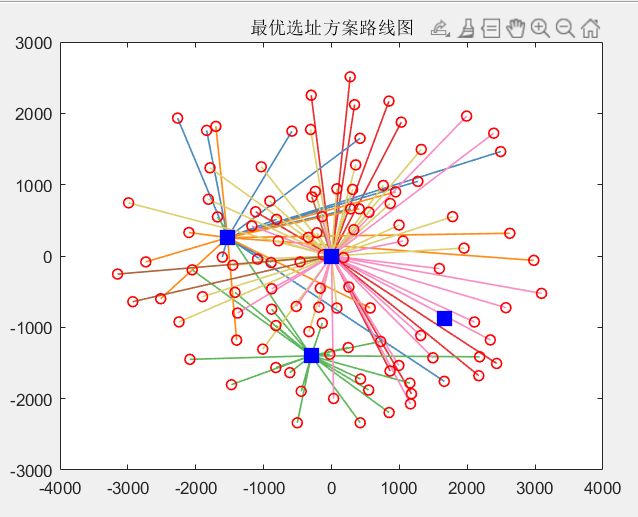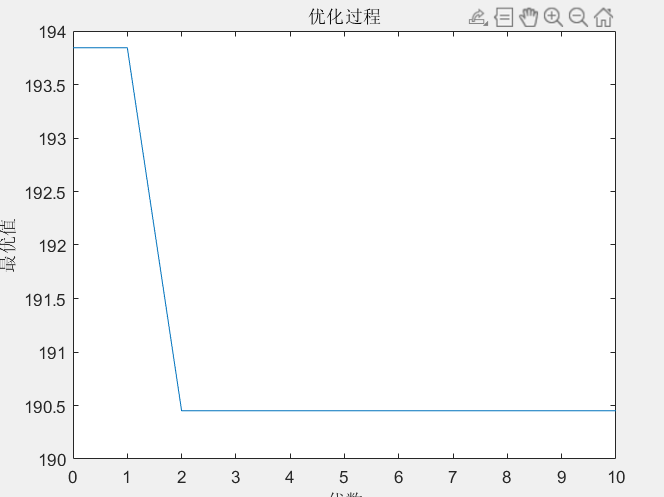选址点1：1->29->28->27->26->25->24->23->22->21->20->1
选址点2：4->33->43->41->40->39->38->37->36->35->34->32->4
选址点3：2->48->62->60->58->56->55->54->53->52->51->50->49->47->76->75->74->73->72->71->70->69->2
选址点4：4->78->90->85->84->83->82->81->80->79->77->4
选址点5：1->92->100->105->108->107->106->104->103->101->2->14->13->12->59->99->86->57->97->96->95->93->9->8->7->5->4->3->1->91->89->88->87->1
选址点6：1->16->11->10->94->1
选址点7：1->19->18->17->15->31->30->46->98->102->6->65->64->63->61->67->68->66->45->44->42->1
最开始的点为选择的配送中心（代码中一共四个备选中心）
如需帮助
VX：zzs1056600403


展开全文• 有哪位大神知道在利用遗传算法求解0-1线性规划的问题时，怎样处理目标函数中的复杂约束条件的问题
• 提出了一种基于量子遗传算法解决多约束 QoS路由问题的算法,详细讨论了该算法用于解决包含带 宽、延时、包丢失率和最小花费等约束条件在内的多约束 QoS路由问题,给出了算法实现的方法和具体流程。实 验结果表明,与...
• 例如采用2进制编码，但是每条染色体等于1的数量只有一个，那么编码0 1 1 0 则不符合条件。两个可行的编码应该怎么交叉保证不会产生不可行解。开发语言
• 针对一类具有约束条件的汽车调度问题，提出了约束性的多种TSP（CMTSP）问题及其模型，给出了一种遗传算法解决。对比实验结果表明：遗传算法具有比启发式算法更好的结果。
• 针对多约束条件的多配送中心有时间窗车辆路径问题，提出了一种二阶段遗传退火算法.在第1阶段，使用遗传算法对客户按供应量和路径长度进行模糊分区；在第2阶段，采用二维变长染色体编码及相应的遗传算子进行混合遗传...
• 设计一种求解约束优化问题的粒子进化变异遗传算法(IGA PSE). 首先, 分析候选解约束条件离差统计信息 与约束违反函数之间的关系及其性质, 基于约束条件离差统计信息提出一种改进约束处理方法; 其次, 基于粒子...
• 约束条件的处理方法 （1）搜索空间限定法 （2）可行解变换法 （3）惩罚函数 遗传算法的高级实现技术 倒位算子 二倍体与显性操作算子 变长度染色体遗传算法 小生境遗传算法 混合遗传算法 并行遗传算法 遗传算法的并行...
声明：本文根据对遗传算法相关资料进行整理所得，所参考出处均在文末进行标注，如有侵权，请联系删除。
算法： 遗传算法
参照书籍： 遗传算法原理及应用（国防工业出版社）
遗传算法的高级实现技术
倒位算子
$\quad$倒 位操作是指颠倒个体编码中随机指定的两个基因座之间的基因排列顺序，从而形成一个新的染色体。
$\quad$具体流程：
（1）随机指定倒位点
（2）以倒位概率$P_{i}$颠倒倒位点之间的基因序列。
例：
$1\quad\vdots\quad1\quad0\quad0\quad0\quad\vdots\quad 1\quad1\quad\rightarrow1\quad\vdots\quad0\quad0\quad0\quad1\quad\vdots\quad1\quad1$
二倍体与显性操作算子
出发点：基因的显性与隐性。
例：
$\begin{array}{l} A b c D e f G \\ a b C D e f G \end{array} \rightarrow A b C D e f G\\$左侧为二倍体结构
$\quad$对于动态系统优化问题，使用单倍体的遗传算法很难达到优化要求，因为对动态环境的变化过程跟踪效果差，使用双倍体效果较好。
变长度染色体遗传算法
$\quad$变长度染色体遗传算法的编码与解码
编码：
$\quad$变长度染色体一般表示方式：$X^m:(i_1,v_1)(i_2,v_2)…(i_k,v_k)…(i_n,v_n)$
$\quad$其中$i_k$为基因编号$v_k$为基因值。
$\quad$例：常规染色体表示：$X:100101$
$\quad$变长度可表示为$X^m:(1,1)(2,0)(3,0)(4,1)(5,0)(6,1)$
$\quad$在变长度染色体遗传算法中，允许长度可长可短。如：
$\quad$$X^m:(1,1)(2,0)(3,0)(4,1)(5,0)(6,1)(3,1)(1,0)$
$\quad$$X^m:(1,1)(3,0)(5,0)(6,1)$
$\quad$前者称为过剩指定，后者称为缺省指定。
解码：
$\quad$（1）在正常指定情况下，将变长度染色体遗传算法中的个体基因型转换为常规遗传算法中的个体基因型。
$\quad$（2）在描述过剩时，取最左边的二元组进行解码。
$\quad$例：$X^m:(1,1)(2,0)(3,0)(4,1)(5,0)(6,1)(3,1)(1,0)$
$\quad$他在常规遗传算法中所对应的个体为：
$\quad$$X：100101$
$\quad$（3）描述不足时，可取规定预先设定的标准值或省缺值。
$\quad$例：$X^m:(1,1)(3,0)(5,0)(6,1)$
$\quad$若省缺值为0，$X:100001$
$\quad$若省缺值为1，$X:110101$
$\quad$切断算子与拼接算子
$\quad$在变长度染色体遗传算法中不使用交叉算子，使用切断算子和拼接算子进行代替。
切断算子：
$\quad$切断算子以某一预先指定的概率，在变长度染色体中随机选择一个基因座，在该处将个体的基因型切断，使之称为两个个体的基因型。
拼接算子：
$\quad$拼接算子以某一预先指定的概率，将两个个体的基因型连接在一起，使他们合并为一个个体的基因型。
$\quad$变长度染色体遗传算法的算法结构
（1）初始化。随机产生M个染色体，长度全部为$k$的个体，以他们作为变长度遗传算法的初始个体集合$P(0)$，其中$k$为根据问题的不同而设定的一个参数，并且$k \le l$。
（2）适应度评价。对变长度的染色体进行解码处理后，评价或计算各个个体的适应度。
（3）基本处理阶段。对群体$P(t)$施加选择算子，以保留适应度较高的个体。
（4）并列处理阶段。对群体$P(t)$施加变异算子、切断算子和拼接算子，以生成新的个体。
（5）重复（2）到（4），直到满足条件为止。
小生境遗传算法
$\quad$在用遗传算法求解多峰值函数的优化计算问题时，经常是只能找到个别的几个最优解，或得到局部最优解，而有时希望优化算法能够找出问题的所有最优解，包括局部最优解和全局最优解。基本遗传算法对此无能为力。在遗传算法中引入小生境的概念，从而解决这类问题，以找出更多的最优解。
$\quad$遗传算法中模拟小生境的方法主要有以下几种：
（1）基于预选择的小生境实现方法
$\quad$基本思想：仅当新生代的子代个体的适应度超过其父代个体适应度时，所产出的子代个体才能替换其父代个体而遗传到下一代群体中，否则父代个体仍保留在下一代群体中。
（2）基于排挤的小生境实现方法
$\quad$基本思想：设置排挤因子$CF$，由群体中随机选取的$1/CF$个个体组成排挤成员，然后依据新产生的个体与排挤成员的相似性来排挤掉一些与排挤成员相类似的个体。个体之间的相似性可用个体编码串之间的海明距离来度量。
（3）基于共享函数的小生境实现方法
$\quad$基本思想：通过反映个体之间相似程度共享函数来调整群体中各个个体的适应度，从而在这以后的群体进化过程中，算法能够依据调整后的适应度来进行选择运算，以维护群体的多样性，创造出小生境的进化环境。
混合遗传算法
$\quad$遗传算法存在问题：容易产生早熟现象、局部寻优能力较差、并不是解决问题的最有效方法、不能避免对同一个可行解多次搜索
$\quad$其他算法：梯度法、爬山法、模拟退火法、列表寻优法具有较强的局部搜索能力。
$\quad$混合遗传算法在标准遗传算法中融合了局部搜索算法的思想。
$\quad$混合算法特点：
（1）引入局部搜索过程。
（2）增加了编码变换操作过程。
$\quad$混合遗传算法的基本构成原则：
（1）尽量采用原有算法的编码方式。
（2）利用原有算法的优点。
（2）改进遗传算子。


展开全文机器学习
• 数降低适应度方法使其满足等式约束条件，但太难收敛或解根本不满足约束条件，因此它是遗传算法的一个瓶颈。根据遗 传算法的性质及等式约束的特点，提出了另一种算法来解决这个瓶颈，并从理论上证明了算法的可行性。...
• ## 遗传算法

万次阅读 多人点赞 2018-06-18 18:02:06
由于本人在研究生期间，许多论文中涉及到优化问题，即给定某个目标函数，以及一些约束条件来求解最优值以及最优解。对于求解这类优化问题，遗传算法是一种不错的选择。接下来，我将分享给大家我对于遗传算法的一些...
由于本人在研究生期间，许多论文中涉及到优化问题，即给定某个目标函数，以及一些约束条件来求解最优值以及最优解。对于求解这类优化问题，遗传算法是一种不错的选择。接下来，我将分享给大家我对于遗传算法的一些拙见，如有不足之处，请大家多多指正。

遗传算法，顾名思义就是根据生物界的遗传进化行为而得名的，其核心思想就是优胜劣汰。

首先让我们先简单回顾一下生物界的遗传进化过程，假设存在某一物种，其种群在一代一代繁衍过程当中，某些个体由于基因突变等因素，导致了变异现象的发生。然而变异也有好坏之分，并非所有的变异都是好的。如果某个个体产生了较好的变异，那么这个个体将会更加能够适应生存环境，因此其生存能力更强，个体更优。相反的如果某个个体产生了不好的变异，那么这个个体必然会被生存环境所淘汰。这便是种群的进化过程。

接下来让我们将生物界中的遗传映射到遗传算法当中。上文中所提到的个体对应于优化问题中的一个解（准确的说是一个解所对应的编码，在下文中会进行讲解），上文中个体的生存能力对应于优化问题中某个解所对应的目标函数值（在遗传算法中称为适应度）。在遗传算法求解过程中，我们也会对个体进行变异等操作，如何判断变异后的个体的好坏呢，自然是根据这个个体的目标函数值（适应度）来进行判断，如果目标函数为最大化问题，那么目标函数值大的个体自然就是好的个体，相反就是不好的个体。

接下来对遗传算法的每一步进行详细说明，但是在此之前需要对一些参数进行说明，这些参数将会运用到遗传算法的过程当中。

种群数量：即种群中个体的数量，此处用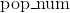来表示

变异概率：用来控制种群中发生变异行为的个体的数量，用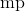表示，取值范围在0~1之间

交叉概率：用来控制种群中发生交叉行为的个体的数量，用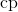表示，取值范围在0~1之间

迭代次数：遗传算法终止的条件，用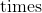表示，即经过多少代的种群迭代之后停止

我们以目标函数最大化为例来讲解遗传算法的具体步骤：

遗传算法总共可以分为6步：1.编码，2.解码，3.求解适应度，4.复制，5.交叉，6.变异

1.编码：遗传算法中的编码可以采用实数编码，以及二进制编码，在这里我们主要介绍二进制编码。假设优化问题的解为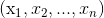，其中每个决策变量对应的的编码长度为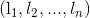，则一个个体的总的编码长度记做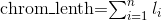，即每个个体被编码为一个长度为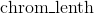的二进制串。由于我们上文中说明了种群数量为，因此，我们在初始化种群时，将对个个体进行随机编码，即生成个长度为的二进制串作为我们的初始种群，其中每一个二进制串为一个个体。

2.解码：解码的目的是为了得到每个个体对应的解的实际值。具体过程如下。首先我们需要取出一个个体中每个决策变量对应的二进制编码段，某个个体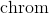的决策变量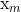对应的编码段为个体的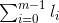位置到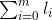位置，即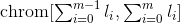（假设个体的下标从0开始，并且设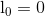），其中包含开始位置对应的二进制位，不包含结束位置对应的二进制位。之后将编码段转换为对应的十进制值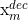。设的取值范围为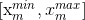，则解码后的实际值为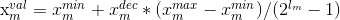。最后我们根据一个个体可以得到一个对应的优化问题的解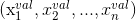，最后对整个种群解码后，得到个解。

3.求解适应度：通过第二步解码后，我们便得到了个解，依次将这些解带入到目标函数，求取出目标函数值便得到了这些解所对应的适应度值，我们记为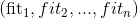。

4.复制：首先，我们可以将适应度最优的个体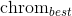找出来，作为复制得到的种群中的第一个个体，作为当前种群最优个体，这个操作也称之为精英保留策略。之后我们再从种群中通过某种选择方案选出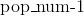个个体，和一开始选出的最优个体组成复制后形成的种群。接下来介绍选择方案，即如何选出个个体。首先通过公式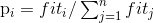计算出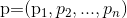，之后再通过公式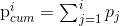计算得出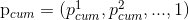。之后我们通过产生一个0到1之间的随机数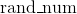，判断位于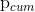的哪个位置，例如，如果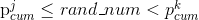，则将原先种群中第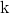个个体选出，如此循环次便得到了选出的个个体。

5.交叉：将种群中的的第一个个体，也就是之前选出的最优个体排除在外不参加交叉操作，其他个个体进行交叉操作。首先有序生成个0到1之间的随机数，记录其中小于交叉概率的随机数的位置，将这些位置对应的个体选出来作为进行交叉操作的个体（在程序编写中，我一般限制选出个体的数量大于零且为偶数，如不符合条件则重新生成随机数进行选择）。之后，从选出的个体中每次按顺序遍历出两个个体进行交叉操作。接下来介绍两个个体之间如何进行交叉操作，设个体起始位下标为0，则个体的最后一位对应的下标为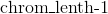。首先产生一个1（包含）到（不包含）之间的随机数，作为这两个个进行体交叉位置，之后将这两个个体在这个位置之后的片段进行交换便完成了交叉操作。依次对之前选出的个体进行此操作，直到被选出的个体全部完成，则种群的交叉操作完成。由于进行了变异操作，种群中最优个体有可能已经发生了变化，因此当完成遍历操作时我们也可以再次进行解码，求适应度，将种群中最优的个体放置在种群的第一个作为新的。

6.变异：首先同交叉操作相同，种群中的第一个个体不进行变异操作，之后的个个体进行变异操作。变异操作的具体方法如下，首先将参加变异操作的个个体连成一条串，其长度为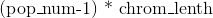，之后一次产生个0到1之间的随机数，记录小于变异概率的随机数的位置，所有小于变异概率的随机数的位置便是这条串上发生变异的位置，通过这个位置可以找到发生变异的个体的，以及在这个个体上的哪个位置发生的变异。例如，某个小于变异概率的随机数在这条串上的位置为，则可以求得发生变异的个体索引为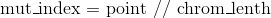，“//”表示整除（向下取整），在这个个体上发生变异的位置为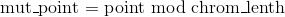，“mod"表示取余。最终确定了变异位置之后，只需观察这个位置上的二进制值是0还是1，如果是0则变异为1，如果是1则变异为0。如此循环直到把所有的发生变异的位置均进行变异操作。最终便产生了新的种群。

最后依次循环执行2至6步来进行种群迭代。

至此，遗传算法的6个步骤都已介绍完毕，需要注意的是，第1步编码操作只在第一次种群迭代时进行，之后的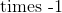次迭代都只执行2至6步。

细心的同学可能会发现，这种方法只适用于目标函数值为正的情况，如果目标函数值既有正值又有负值则在复制操作时会出问题，那么我的解决方法是如果目标函数既有正值又有负值，因为目标函数是求取最大化，所以负值所对应的个体应该被舍弃，所以所有适应度为负值的个体将其适应度设置为0，这个问题便得以解决。也许又会有同学问，如果目标函数值是小于0的呢？确实，目标函数值小于0在当前的复制操作也会出现问题，因此，可以对目标函数加负号，这样目标函数值便为正，并且原问题如果是最小化问题，则现在转化为了最大化问题，这个问题便得以解决。但是如果原问题是最大化问题，加负号之后虽然目标函数值为正，但是转为了最小化问题，此时我们可以采取取倒数转化为最大化问题，同样可以解决这个问题。

到这里，遗传算法的基本原理已经讲完，由于这也是本人的第一篇博客，所以可能存在许多不足，也请大家多多包涵，多多指正，希望对正在学习遗传算法的你有帮助！

以下附上本人使用python编写的遗传算法的相关程序以供大家参考

from numpy import random as rd
import copy
import numpy as np
import matplotlib.pyplot as plt
import time
np.set_printoptions(suppress=True, linewidth=1000)

def test_func(x1, x2):
# 这是一个用于测试的目标函数
return 1 / (x1 ** 2 + x2 ** 2 + 0.5)

def GetTime(func_name):
def inner(*args, **kwargs):
start_time = time.time()
ret = func_name(*args, **kwargs)
end_time = time.time()
run_time = end_time - start_time
print("遗传算法迭代用时%f秒" % run_time)
return ret
return inner

class GA(object):

def __init__(self, pop_num, iter_time, cp, mp, coding_way, var_code_lenth, objective_function, **kwargs):
"""
:param pop_num: 种群数量
:param iter_time: 迭代次数
:param cp: 交叉概率
:param mp: 变异概率
:param coding_way: 编码方式，传入"b"为二进制编码，传入"r"为实数编码
:param var_code_lenth: 每个决策变量的编码长度
:param objective_function: 传入自定义的计算目标函数值得函数对象, 函数对象在定义时需要设置决策变量为传入参数，
决策变量参数的传入顺序需与**kwargs决策变量一一对应， 例如def objective_func(x1, x2, x3),
在**kwargs传入时应该以x1=(x1_min, x1_max), x2=(x2_min, x2_max), x3=(x3_min, x3_max)的顺序传入
并且目标函数最终返回变量带入后求得的结果, 要求目标函数求最大值,一定要注意目标函在给定的定义域内不全小于等于0
:param kwargs: 传入方式为：决策变量1 = (决策变量1的最大值, 决策变量1的最小值), 决策变量2 = (决策变量2的最大值, 决策变量2的最小值), ...
"""
self.pop_num = pop_num
self.iter_time = iter_time
self.cp = cp
self.mp = mp
self.coding_way = coding_way.lower()
self.var_code_lenth = var_code_lenth
self.obj_func = objective_function
self.kwargs = kwargs
# self.chrom_lenth为每条染色体（每个个体）编码长度
self.chrom_lenth = len(self.kwargs) * self.var_code_lenth
for min_, max_ in kwargs.values():
assert min_ < max_, "参数填写有误，最小值不能大于最大值"
# self.best_fitness_value用于存放每一代种群中最优的适应度，迭代结束后通过self.best_fitness_value[-1]取出最终的最优适应度值
# self.best_value用于存放每一代种群中最优适应度对应的个体解码值，也就是决策变量的值，迭代结束后通过self.best_value[-1]取出最优适应度对应的决策变量
self.best_fitness_value = []
self.best_value = []
try:
slices = tuple([slice(value_range, value_range, 300j) for value_range in self.kwargs.values()])
var_values = np.mgrid[slices]
if np.all(objective_function(*var_values) <= 0):
exit("目标函数值域小于等于0，请将目标函数值域转换为大于等于0(对目标函数取负号，注意取负号后原目标求最大则转为了求最小，可以在采取取倒数转为求最大)")
except Exception as e:
pass

def code_(self):
# 编码
while True:
if self.coding_way == "b":
self.chromsoms = rd.randint(0, 2, (self.pop_num, self.chrom_lenth))
else:
self.chromsoms =[((("%"+"."+str(self.var_code_lenth)+"f,") * len(self.kwargs)) % tuple(i)).strip(",").split(",")\
for i in rd.random((self.pop_num, len(self.kwargs)))]
self.decode_()
if not np.all(np.array(self.get_fitness_value()) <= 0):
break

def decode_(self):
# 解码
# self.values用于存放解码后的变量值
self.values = []
if self.coding_way == "b":
for chrom in self.chromsoms:
# value用于每个个体解码后的变量值，解码规则：变量最小值 + （变量最大值 - 变量最小值）/ （2 ** 单个变量编码长度 - 1） * 变量实际编码转换为10进制的值
# len(value)应该与决策变量个数相同
value = []
for j in range(len(self.kwargs)):
var_min, var_max = list(self.kwargs.values())[j]
var_chrom = "".join([str(i) for i in chrom[j * self.var_code_lenth:(j + 1) * self.var_code_lenth]])
var_bin_value = int(var_chrom, 2)
bin_value = 2 ** self.var_code_lenth - 1
var_value = var_min + var_bin_value * (var_max - var_min) / bin_value
value.append(var_value)
self.values.append(value)
else:
for chrom in self.chromsoms:
value = []
for i in range(len(chrom)):
var_min, var_max = list(self.kwargs.values())[i]
value.append(var_min + float(chrom[i]) * (var_max - var_min))
self.values.append(value)

def get_fitness_value(self):
# 求适应度
# self.fit_values用于存放每个个体的适应度，即目标函数值
self.fit_values = []
for value in self.values:
if self.obj_func(*tuple(value)) < 0:
self.fit_values.append(0)
else:
self.fit_values.append(self.obj_func(*tuple(value)))
return self.fit_values

def copy_(self):
# 复制操作
best_chrom_index = self.fit_values.index(max(self.fit_values))
self.best_value.append(copy.deepcopy(self.values[best_chrom_index]))
self.best_fitness_value.append(self.fit_values[best_chrom_index])
chromsoms = [copy.deepcopy(self.chromsoms[best_chrom_index])]
p = np.array(self.fit_values) / np.sum(self.fit_values)
p_cum = np.cumsum(p)
for i in range(self.pop_num - 1):
rand_num = rd.random()
if rand_num < p_cum:
chromsoms.append(copy.deepcopy(self.chromsoms))
continue
for k in range(self.pop_num - 1):
if p_cum[k] <= rand_num < p_cum[k + 1]:
chromsoms.append(copy.deepcopy(self.chromsoms[k + 1]))
break
self.chromsoms = chromsoms

def crossover(self):
while True:
cros_index = []
for i in range(1, self.pop_num):
rand_num = rd.random()
if rand_num < self.cp:
cros_index.append(i)
if cros_index and len(cros_index) % 2 == 0:
break
if self.coding_way == "b":
for i in range(0, len(cros_index), 2):
cros_point = rd.randint(1, self.chrom_lenth)
a = copy.deepcopy(self.chromsoms[cros_index[i]][cros_point:])
self.chromsoms[cros_index[i]][cros_point:] = copy.deepcopy(self.chromsoms[cros_index[i + 1]][cros_point:])
self.chromsoms[cros_index[i + 1]][cros_point:] = a
else:
for i in range(0, len(cros_index), 2):
cros_point = rd.randint(3, self.var_code_lenth + 2, len(self.kwargs))
for k in range(len(self.kwargs)):
a = "".join(list(self.chromsoms[cros_index[i]][k])[cros_point[k]:])
self.chromsoms[cros_index[i]][k] = self.chromsoms[cros_index[i]][k][:cros_point[k]] + self.chromsoms[cros_index[i + 1]][k][cros_point[k]:]
self.chromsoms[cros_index[i + 1]][k] = self.chromsoms[cros_index[i + 1]][k][:cros_point[k]] + a

def mutate(self):
self.decode_()
self.get_fitness_value()
best_chrom_index = self.fit_values.index(max(self.fit_values))
# 精英保留
chromsoms = [copy.deepcopy(self.chromsoms[best_chrom_index])]
# 排除精英，剩下的个体进行变异操作
self.chromsoms.pop(best_chrom_index)
while True:
mutate_point = []
for i in range(self.chrom_lenth * len(self.chromsoms)):
if rd.random() < self.mp:
mutate_point.append(i)
if mutate_point:
break
if self.coding_way == "b":
for i in mutate_point:
mutate_index = i // self.chrom_lenth
mutate_point = i % self.chrom_lenth
if self.chromsoms[mutate_index][mutate_point] == 0:
self.chromsoms[mutate_index][mutate_point] = 1
else:
self.chromsoms[mutate_index][mutate_point] = 0
else:
for i in mutate_point:
mutate_index = i // self.chrom_lenth
mutate_point = i % self.chrom_lenth
mutate_var_index = mutate_point // self.var_code_lenth
mutate_var_point = mutate_point % self.var_code_lenth
a = copy.deepcopy(self.chromsoms[mutate_index])
while True:
self.chromsoms[mutate_index][mutate_var_index] = self.chromsoms[mutate_index][mutate_var_index][:2 + mutate_var_point] + str(rd.randint(0, 10)) + self.chromsoms[mutate_index][mutate_var_index][mutate_var_point + 3:]
if self.chromsoms[mutate_index] != a:
break
chromsoms.extend(self.chromsoms)
self.chromsoms = chromsoms

@GetTime
def run(self):
"""
如果目标函数极值求解没有约束条件，则可直接调用run来进行求解
否则需要自己调用code_, decode_, get_fitness_value, copy_,
crossover, mutate方法进行迭代
"""
self.code_()
for i in range(self.iter_time):
if i == 0:
print("遗传算法开始迭代...")
if i % int(0.15 * self.iter_time) == 0:
print("遗传算法迭代第%d代" % i)
self.decode_()
self.get_fitness_value()
self.copy_()
self.crossover()
self.mutate()
print("最优适应度：", self.best_fitness_value[-1])
print("最优个体：", self.best_value[-1])
self.draw()
return self.best_fitness_value[-1], self.best_value[-1]

def draw(self):
fig = plt.figure()
ax = plt.subplot(1, 1, 1)
ax.plot(np.arange(1, self.iter_time + 1), self.best_fitness_value, color="r", label="fitness value")
ax.set_xlabel("generations")
ax.set_ylabel("fitness value")
if self.coding_way == "r":
ax.set_title("real number coding GA")
else:
ax.set_title("binary coding GA")
ax.legend()
plt.show()

def main():
print("########二进制编码##############")
ga_b = GA(200, 200, 0.75, 0.02, "b", 20, test_func, x1=(-30, 30), x2=(-10, 22))
ga_b.run()
print("########实数编码################")
ga_r = GA(200, 200, 0.75, 0.02, "r", 8, test_func, x1=(-30, 30), x2=(-10, 22))
ga_r.run()

if __name__ == "__main__":
main()



展开全文• 使用贪婪交叉算子、自适应变异算子和带有精英保留策略的选择算子相结合对基本遗传算法进行了改进，针对实际TSP中的约束条件讨论了罚方法在遗传算法中的应用，提出了自适应的惩罚函数，并将其与改进后的遗传算法相...旅行商问题 罚函数
• 遗传算法求解带约束优化问题（源码实现）。方法1：开始设计编码规则时，让解编码就只可能在可行区域内。方法2：设计合理的交叉算子和变异算子，使得满足这些算子本身的特性的前提下，还让算子运算后的染色体也在可行...
• 例如，Nelder-Mead、遗传算法和差分进化，以及模拟退火. 直接搜索方法往往收敛速度较慢，但可以对函数和约束条件中噪声存在的限制更为宽松. 通常情况下，算法只建立问题的局部模型. 此外，许多这种算法总是寻求目标...人工智能
• 3.7 约束条件的处理方法 3.8 遗传算法工具箱 第四章 遗传算法的高级实现技术 4.1 倒位算子 4.2 二倍体与显性操作算子 4.3 变长度染色体遗传算法 4.4 小生境遗传算法 4.5 混合遗传算法 第五章 并行遗传算法 ...
• 根据铜板带生产流程的特点，考虑...该算法采用矩阵编码、混合选择策略和三个体交叉方法，使其在有限的步骤内，找出所有满足约束条件的最优或次优解。仿真结果表明该算法可以有效地解决铜板带生产调度问题，具有实用性。
• 利用多目标法处理约束条件，提出一种改进的基于多目标优化的遗传算法用于求解约束优化问题。该算法将约束优化问题转化为两个目标的多目标优化问题; 利用庄家法构造非劣个体，将种群分为支配子种群和非支配子种群，以...
• 利用多目标法处理约束条件，提出一种改进的基于多目标优化的遗传算法用于求解约束优化问题。该算法将约束优化问题转化为两个目标的多目标优化问题; 利用庄家法构造非劣个体，将种群分为支配子种群和非支配子种群，以...
• matlab语言编写flying-V型仓库布局的拣货路径优化方法，主要是遗传算法，模拟退火算法，蚁群算法等，本文主要讲述基于遗传算法结合模拟退火算法进行优化的仿真实验。 仓库布局为flying-V型宽道模式，即不考虑拣货...matlab
• 针对油田开发钻井剖面地层对比的具体特点和实际问题应满足的约束条件，提出了一种基于目标约束遗传算法的地层对比方法。该方法既能较好地完成常规地层对比，也可对因断层或地层尖灭等地质现象造成的地层缺失、错位等...
• 4.7 约束条件的处理 第五章 遗传算法工具箱函数 5.1 工具箱结构 5.1.1 种群表示和初始化 5.1.2 适应度计算 5.1.3 选择函数 5.1.4 交叉算子 5.1.5 变异算子 5.1.6 多子群支持 5.2 遗传算法中的通用函数 5.2.1 函数 ......